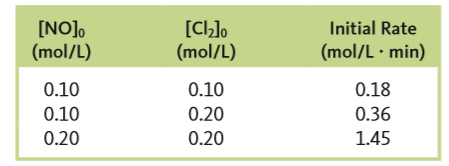# Problem: The reaction2NO (g) + Cl2 (g) → 2NOCl (g)was studied at -10°C. The following results were obtained whereRate = -Δ[Cl2] / Δta. What is the rate law?

###### FREE Expert Solution

Recall that the rate law only focuses on the reactant concentrations and has a general form of:

k = rate constant
A & B = reactants
x & y = reactant orders

96% (399 ratings)###### Problem Details

The reaction

2NO (g) + Cl2 (g) → 2NOCl (g)

was studied at -10°C. The following results were obtained where

Rate = -Δ[Cl2] / Δta. What is the rate law?